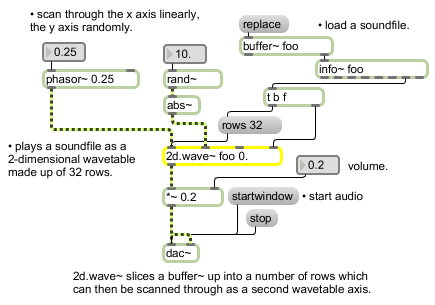# 2d.wave~

Two-dimensional wavetable

## Description

2d.wave~ is similar to wave~, but with an additional axis. A given ms range of an audio file will be divided into n rows. Y phase input will determine which row(s) will be used for playback. Try loading a 16-bar loop and setting your rows to be 16 (or 7 for that matter). Try also using a short (150 ms or so) section of an audio file as a 2-d wave table. Set the phasor of one axis to be very fast, and one to be very slow.

## Arguments

Name Type Opt Description
buffer-object-name symbol Obligatory. Names the buffer~ object whose sample memory is used by 2d.wave~ for its stored waveform. Note that if the underlying data in a buffer~ changes, the signal output of 2d.wave~ will change, since it does not copy the sample data in a buffer~. 2d.wave~ always uses the first n channels of a multi-channel buffer~, where n is the number of the 2d.wave~ object's output channels. The default number of channels, set by the third argument to the 2d.wave~ object, is 1.
start and end-points float or int opt After the buffer~ name argument, you can type in values for the start and end points of the waveform, as millisecond offsets from the beginning of a buffer~ object's sample memory. By default the start point is 0 and the end point is the end of the sample. If you want to set a non-zero start point but retain the sample end as the waveform end point, use only a single typed-in argument after the buffer~ name. If a signal is connected to the start point (middle) inlet, the initial waveform start point argument is ignored. If a signal is connected to the end point (right) inlet, the initial waveform end point is ignored. The number of channels in the buffer~ file and the number of rows to be used may also be specified.
number-of-output-channels int opt Sets the number of output channels, which determines the number of outlets that the 2d.wave~ object will have. The maximum number of channels is 8. The default is 1. If the audio file being played has more output channels than the 2d.wave~ object, higher-numbered channels will not be played. If the audio file has fewer channels, the signals coming from the extra outlets of 2d.wave~ will be 0.

## Messages

 int start and end-points [int] In 3rd or 4th inlets: Numbers can be used instead of signal objects to control the start and end points of the waveform, provided a signal is not connected to the inlet that receives the number. float start and end-points [float] In 3rd or 4th inlets: Numbers can be used instead of signal objects to control the start and end points of the waveform, provided a signal is not connected to the inlet that receives the number. (mouse) Double-clicking on the 2d.wave~ object will open a window that displays the audio file loaded in the buffer associated with the object. set buffer-name [symbol]start-point [float]end-point [float] The word set, followed by a symbol, sets the buffer~ used by 2d.wave~ for its stored waveform. The symbol can optionally be followed by two values setting new waveform start and end points. If the values are not present, the default start and end points (the start and end of the sample) are used. If signal objects are connected to the start and/or end point inlets, the start and/or end point values are ignored. rows number-of-divisions [int] The word rows, followed by an int, sets the number of rows a given range of an audio file will be divided into. The phase input signal value received in the 2nd inlet of 2d.wave~ determines which row(s) are used for playback. The default value is 0. signal In left inlet: Input signal values progressing from 0 to 1 are used to scan a specified range of samples in a buffer~ object. The output of a phasor~ can be used to control 2d.wave~ as an oscillator, treating the range of samples in the buffer~ as a repeating waveform. However, note that when changing the frequency of a phasor~ connected to the left inlet of 2d.wave~, the perceived pitch of the signal coming out of 2d.wave~ may not correspond exactly to the frequency of phasor~ itself if the stored waveform contains multiple or partial repetitions of a waveform. You can invert the phasor~ to play the waveform backwards. In 2nd inlet: Input signal values progressing from 0 to 1 are used to determine which of the row(s) specified by the rows message will be used for playback. You can invert the phasor~ to reverse the order in which rows are played. In 3rd inlet: The start of the waveform as a millisecond offset from the beginning of a buffer~ object's sample memory. In 4th inlet: The end of the waveform as a millisecond offset from the beginning of a buffer~ object's sample memory.

## Output

signal: The portion of the buffer~ specified by the 2d.wave~ object's start and end points is scanned by signal values ranging from 0 to 1 in the 2d.wave~ object's inlet, and the corresponding sample value from the buffer~ is sent out the 2d.wave~ object's outlet. If the signal received in the object's inlet is a repeating signal such as a sawtooth wave from a phasor~, the resulting output will be a waveform (excerpted from the buffer~) repeating at the frequency corresponding to the repetition of the input signal.

## ExamplesLoop through part of a sample treating it as a variable-size wavetable